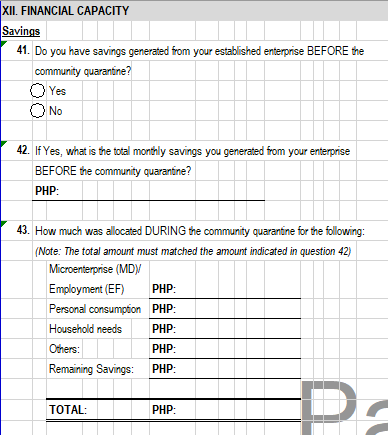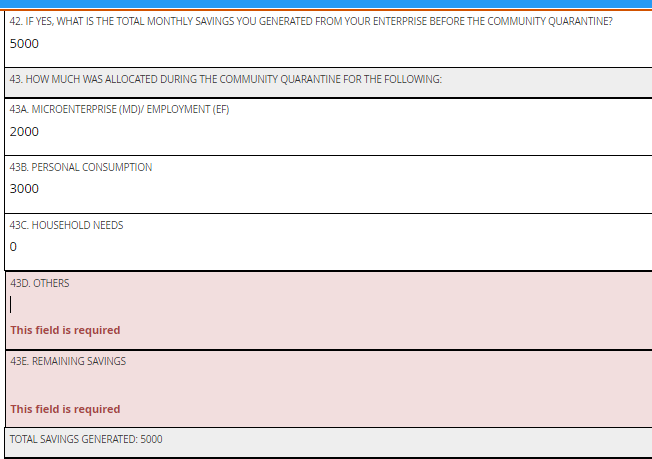# How to constraint a sum so that the inputs do not exceed the total entered previously?

I want the total sum to be matched with my question “Q”. meaning, if the figures they inputs in Q1 to Q5 already matched the amount in the question “Q”, they cannot write any figures.

Isavings calculation.xlsx (9.1 KB)

@Kal_Lam it should be like this.You could do this as outlined in the post discussed previously:

Thank you so much @Kal_Lam . it was perfect!

1 Like

can we disable the items if the total of the other items reached the indicated amount from the previous question? meaning, if the first two items among the five reached the total amount already, they cannot encode in the last three items.In this case, you could do this as outlined in the image shared below:

#### Reference xlsform:

Constraint Example.xlsx (10.7 KB)

Kal
Thank you for this help. I am not able to get it. Please help… My requirement is answer to second question should be less than or equal to first question. I am not authorised to upload a file. hence typing it here

type name label required contraint relevant calculation
start start
end end
decimal total Total land true
decimal kharif kharif true .>=0 and .<=\${total} \${total} > 0
calculate version ‘vgM5PJ3vQpqaoxkLuxZj6V’

Kal,
@bhaktharvali, you missed to update your `relevant` column as shown in the image above. Update the same and your xlsform should also work.